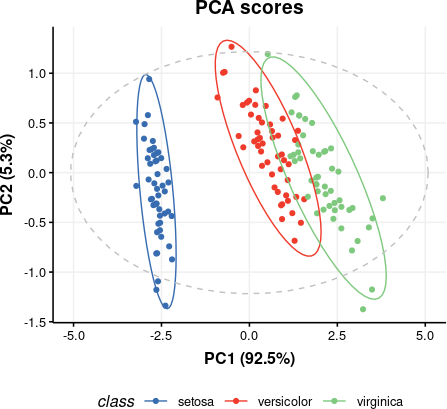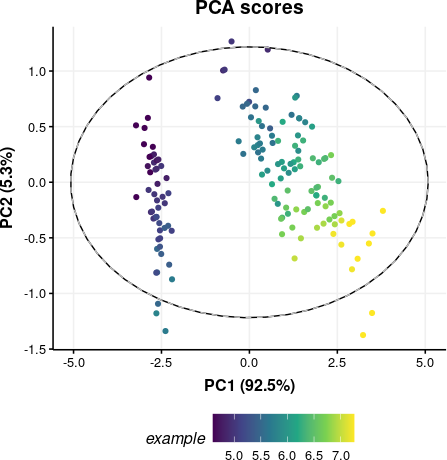# 1 Introduction

The ‘structToolbox’ includes an extensive set of data (pre-)processing and analysis tools for metabolomics and other omics, with a strong emphasis on statistics and machine learning. The methods and tools have been implemented using class-based templates available via the `struct` (Statistics in R Using Class-based Templates) package. The aim of this vignette is to introduce the reader to basic and more advanced structToolbox-based operations and implementations, such as the use of `struct` objects, getting/setting methods/parameters, and building workflows for the analysis of mass spectrometry (MS) and nuclear magnetic resonance (NMR)-based Metabolomics and proteomics datasets. The workflows demonstrated here include a wide range of methods and tools including pre-processing such as filtering, normalisation and scaling, followed by univariate and/or multivariate statistics, and machine learning approaches.

# 2 Getting started

The latest version of `structToolbox` compatible with your current R version can be installed using `BiocManager`.

``````# install BiocManager if not present
if (!requireNamespace("BiocManager", quietly = TRUE))
install.packages("BiocManager")

# install structToolbox and dependencies
BiocManager::install("structToolbox")``````

A number of additional packages are needed for this vignette.

``````## install additional bioc packages for vignette if needed
#BiocManager::install(c('pmp', 'ropls', 'BiocFileCache'))

## install additional CRAN packages if needed
#install.packages(c('cowplot', 'openxlsx'))

suppressPackageStartupMessages({
# Bioconductor packages
library(structToolbox)
library(pmp)
library(ropls)
library(BiocFileCache)

# CRAN libraries
library(ggplot2)
library(gridExtra)
library(cowplot)
library(openxlsx)
})

# use the BiocFileCache

# 3 Introduction to `struct` objects, including models, model sequences, model charts and ontology.

## 3.1 Introduction

PCA (Principal Component Analysis) and PLS (Partial Least Squares) are commonly applied methods for exploring and analysing multivariate datasets. Here we use these two statistical methods to demonstrate the different types of `struct` (STatistics in R Using Class Templates) objects that are available as part of the `structToolbox` and how these objects (i.e. class templates) can be used to conduct unsupervised and supervised multivariate statistical analysis.

## 3.2 Dataset

For demonstration purposes we will use the “Iris” dataset. This famous (Fisher’s or Anderson’s) dataset contains measurements of sepal length and width and petal length and width, in centimeters, for 50 flowers from each of 3 class of Iris. The class are Iris setosa, versicolor, and virginica. See here (https://stat.ethz.ch/R-manual/R-devel/library/datasets/html/iris.html) for more information.

Note: this vignette is also compatible with the Direct infusion mass spectrometry metabolomics “benchmark” dataset described in Kirwan et al., Sci Data 1, 140012 (2014) (https://doi.org/10.1038/sdata.2014.12).

Both datasets are available as part of the structToolbox package and already prepared as a `DatasetExperiment` object.

``````## Iris dataset (comment if using MTBLS79 benchmark data)
D = iris_DatasetExperiment()
D\$sample_meta\$class = D\$sample_meta\$Species

## MTBLS (comment if using Iris data)
# D = MTBLS79_DatasetExperiment(filtered=TRUE)
# M = pqn_norm(qc_label='QC',factor_name='sample_type') +
#   knn_impute(neighbours=5) +
#   glog_transform(qc_label='QC',factor_name='sample_type') +
#   filter_smeta(mode='exclude',levels='QC',factor_name='sample_type')
# M = model_apply(M,D)
# D = predicted(M)

# show info
D``````
``````## A "DatasetExperiment" object
## ----------------------------
## name:          Fisher's Iris dataset
## description:   This famous (Fisher's or Anderson's) iris data set gives the measurements in centimeters of
##                  the variables sepal length and width and petal length and width,
##                  respectively, for 50 flowers from each of 3 species of iris. The species are
##                  Iris setosa, versicolor, and virginica.
## data:          150 rows x 4 columns
## sample_meta:   150 rows x 2 columns
## variable_meta: 4 rows x 1 columns``````

### 3.2.1 DatasetExperiment objects

The `DatasetExperiment` object is an extension of the `SummarizedExperiment` class used by the Bioconductor community. It contains three main parts:

1. `data` A data frame containing the measured data for each sample.
2. `sample_meta` A data frame of additional information related to the samples e.g. group labels.
3. `variable_meta` A data frame of additional information related to the variables (features) e.g. annotations

Like all `struct` objects it also contains `name` and `description` fields (called “slots” is R language).

A key difference between `DatasetExperiment` and `SummarizedExperiment` objects is that the data is transposed. i.e. for `DatasetExperiment` objects the samples are in rows and the features are in columns, while the opposite is true for `SummarizedExperiment` objects.

All slots are accessible using dollar notation.

``````# show some data
``````##   Sepal.Length Sepal.Width Petal.Length Petal.Width
## 1          5.1         3.5          1.4         0.2
## 2          4.9         3.0          1.4         0.2
## 3          4.7         3.2          1.3         0.2
## 4          4.6         3.1          1.5         0.2
## 5          5.0         3.6          1.4         0.2
## 6          5.4         3.9          1.7         0.4``````

## 3.3 Using `struct` model objects

### 3.3.1 Statistical models

Before we can apply e.g. PCA we first need to create a PCA object. This object contains all the inputs, outputs and methods needed to apply PCA. We can set parameters such as the number of components when the PCA model is created, but we can also use dollar notation to change/view it later.

``````P = PCA(number_components=15)
P\$number_components=5
P\$number_components``````
``##  5``

The inputs for a model can be listed using `param_ids(object)`:

``param_ids(P)``
``##  "number_components"``

Or a summary of the object can be printed to the console:

``P``
``````## A "PCA" object
## --------------
## name:          Principal Component Analysis (PCA)
## description:   PCA is a multivariate data reduction technique. It summarises the data in a smaller number of
##                  Principal Components that maximise variance.
## input params:  number_components
## predicted:     that
## seq_in:        data``````

### 3.3.2 Model sequences

Unless you have good reason not to, it is usually sensible to mean centre the columns of the data before PCA. Using the `STRUCT` framework we can create a model sequence that will mean centre and then apply PCA to the mean centred data.

``M = mean_centre() + PCA(number_components = 4)``

In `structToolbox` mean centring and PCA are both model objects, and joining them using “+” creates a model_sequence object. In a model_sequence the outputs of the first object (mean centring) are automatically passed to the inputs of the second object (PCA), which allows you chain together modelling steps in order to build a workflow.

The objects in the model_sequence can be accessed by indexing, and we can combine this with dollar notation. For example, the PCA object is the second object in our sequence and we can access the number of components as follows:

``M\$number_components``
``##  4``

### 3.3.3 Training/testing models

Model and model_sequence objects need to be trained using data in the form of a `DatasetExperiment` object. For example, the PCA model sequence we created (`M`) can be trained using the iris `DatasetExperiment` object (‘D’).

``M = model_train(M,D)``

This model sequence has now mean centred the original data and calculated the PCA scores and loadings.

Model objects can be used to generate predictions for test datasets. For the PCA model sequence this involves mean centring the test data using the mean of training data, and the projecting the centred test data onto the PCA model using the loadings. The outputs are all stored in the model sequence and can be accessed using dollar notation. For this example we will just use the training data again (sometimes called autoprediction), which for PCA allows us to explore the training data in more detail.

``M = model_predict(M,D)``

Sometimes models don’t make use the training/test approach e.g. univariate statsitics, filtering etc. For these models the `model_apply` method can be used instead. For models that do provide training/test methods, `model_apply` applies autoprediction by default i.e. it is a short-cut for applying `model_train` and `model_predict` to the same data.

``M = model_apply(M,D)``

The available outputs for an object can be listed and accessed like input params, using dollar notation:

``output_ids(M)``
``````##  "scores"      "loadings"    "eigenvalues" "ssx"         "correlation"
##  "that"``````
``M\$scores``
``````## A "DatasetExperiment" object
## ----------------------------
## name:
## description:
## data:          150 rows x 4 columns
## sample_meta:   150 rows x 2 columns
## variable_meta: 4 rows x 1 columns``````

### 3.3.4 Model charts

The `struct` framework includes chart objects. Charts associated with a model object can be listed.

``chart_names(M)``
``````##  "pca_biplot"           "pca_correlation_plot" "pca_dstat_plot"

Like model objects, chart objects need to be created before they can be used. Here we will plot the PCA scores plot for our mean centred PCA model.

``````C = pca_scores_plot(factor_name='class') # colour by class
chart_plot(C,M)``````Note that indexing the PCA model is required because the `pca_scores_plot` object requires a PCA object as input, not a model_sequence.

If we make changes to the input parameters of the chart, `chart_plot` must be called again to see the effects.

``````# add petal width to meta data of pca scores
M\$scores\$sample_meta\$example=D\$data[,1]
# update plot
C\$factor_name='example'
chart_plot(C,M)``````The `chart_plot` method returns a ggplot object so that you can easily combine it with other plots using the `gridExtra` or `cowplot` packages for example.

``````# scores plot
C1 = pca_scores_plot(factor_name='class') # colour by class
g1 = chart_plot(C1,M)

# scree plot
C2 = pca_scree_plot()
g2 = chart_plot(C2,M)

# arange in grid
grid.arrange(grobs=list(g1,g2),nrow=1)``````